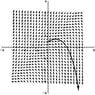# Differential Equations in Mathematica

• Mathematica
amcavoy
I know how to solve them in Mathematica, but is there a way I can plot slope fields / integral curves?

Homework Helper
apmcavoy said:
I know how to solve them in Mathematica, but is there a way I can plot slope fields / integral curves?
yes there is

amcavoy
Well, how would I do it?

Homework Helper
There is a standard package
GraphicsPlotField
all you need for particular curves is to define a function using DSolve or NDSolve
f[x_,x0_,y0_]:=NDSolve[{y'[x]+y[x]==0,y[x0]==y0},y[x],{x,y0,10}][1,1,2]
for the slope field use the DE to get the slope

Homework Helper
Hey Apmcavoy, can you follow this (note the double equal signs):

Code:
<<GraphicsPlotField
<<GraphicsArrow

sol1=NDSolve[{y'[x]==y[x]-x,y==0.5},y,{x,0,3}];
fsol[x_]:=Evaluate[y[x]/.Flatten[sol1]];
xpt=2.6
xed=2.7
ypt=fsol[2.6]
yed=fsol[2.7]
a1=Graphics[Arrow[{xpt,ypt},{xed,yed}]];

pv=PlotVectorField[{1,y-x},{x,-3,3},{y,-3,3},PlotRange->{{-4,4},{-4,4}},
PlotPoints->25,Axes->True]
pt1=Plot[fsol[x],{x,0,2.7},PlotStyle->{{Thickness[0.01]}}]
Show[{pv,pt1,a1}]

A plot of the results is attached. If so, can you post the same for your differential equation?

Edit: Alright, you don't need some of that stuf: arrow tip at the end of the curve, the axes->True, thickness, plotstyle, plotpoints. Just take them out to cut it down.

#### Attachments

•slope field.JPG
26.3 KB · Views: 422
Last edited:
amcavoy
I'm not on my home computer now, but I will post it tomorrow most likely. I would have thought Wolfram would have a quicker way to do this, but I guess this is it. Thanks a lot saltydog for the information! I will try that out as soon as possible.

Homework Helper
apmcavoy said:
I'm not on my home computer now, but I will post it tomorrow most likely. I would have thought Wolfram would have a quicker way to do this, but I guess this is it. Thanks a lot saltydog for the information! I will try that out as soon as possible.

Code:
<<GraphicsPlotField

sol1=NDSolve[{y'[x]==y[x]-x,y==0.5},y,{x,0,3}];
pt1=Plot[Evaluate[y[x]/.sol1]

pv=PlotVectorField[{1,y-x},{x,-3,3},{y,-3,3}]

Show[{pt1,pv}]

amcavoy
The longer way worked great. However, I couldn't get the cut-down version to work properly. Any ideas why? Thanks saltydog.

Homework Helper
apmcavoy said:
The longer way worked great. However, I couldn't get the cut-down version to work properly. Any ideas why? Thanks saltydog.

Alright I'm sorry. That's what I get for posting it without trying it first. Some typos: (no x range in Plot). Also the <<Graphics line should be in it's own cell as it needs to be executed only once to load the package (PlotField is a library of functions).

Code:
<<GraphicsPlotField

sol1=NDSolve[{y'[x]==y[x]-x,y==0.5},y,{x,0,3}];
pt1=Plot[Evaluate[y[x]/.sol1,{x,0,3}]

pv=PlotVectorField[{1,y-x},{x,-3,3},{y,-3,3}]

Show[{pt1,pv}]